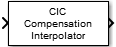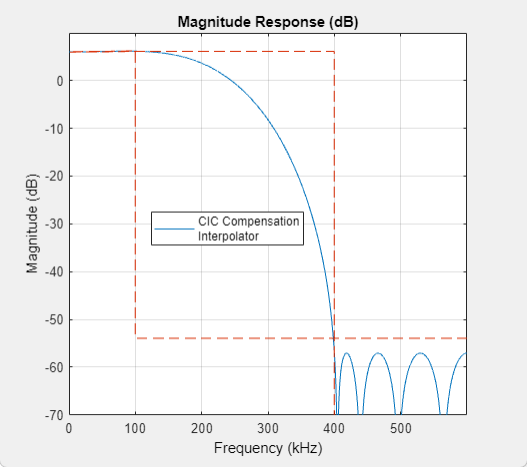# CIC Compensation Interpolator

Compensate for CIC filter using FIR interpolator

## Library

Filtering/Filter Designs

`dspfdesign`

•## Description

The CIC Compensation Interpolator block uses an FIR polyphase interpolator as the compensation filter. CIC compensation interpolators are multirate FIR filters that can be cascaded with CIC interpolators to mitigate the drawbacks of the CIC filters.

CIC interpolation filters are used in areas that require high interpolation. These filters are popular in ASICs and FPGAs, since they do not have any multipliers. CIC filters have two drawbacks:

• CIC filters have a magnitude response that causes a droop in the passband region. This magnitude response is:

$abs{\left(\frac{\mathrm{sin}\left(M\frac{\omega }{2}\right)}{\mathrm{sin}\left(\frac{\omega }{2}\right)}\right)}^{n}$

• M — Differential delay

• n — Number of stages

• ω — Normalized angular frequency

• CIC filters have a wide transition region.

The compensation interpolator filters have an inverse sinc passband response to correct for the CIC droop, and they have a narrow transition width.

This block brings the capabilities of the `dsp.CICCompensationInterpolator` System object™ to the Simulink® environment.

## Dialog Box

### Main TabRate change factor

Rate change factor for the CIC filter to be compensated, specified as a positive scalar integer. The default is `2`.

Number of sections

Number of integrator and comb sections of the CIC filter to be compensated, specified as a positive scalar integer. The default is `2`.

Differential delay

Delay value used in each of the comb sections of the CIC filter to be compensated, specified as a positive scalar integer. The default is `1`.

Filter parameters
Interpolation factor

Interpolation factor of the compensator, specified as a positive scalar integer. The default value is `2`.

Minimum order filter design

When you select this check box, the block designs filters with the minimum order that meets the specifications for passband frequency, stopband frequency, passband ripple, and stopband attenuation. When you clear this check box, the block designs filters with the order that you specify in Filter order.

By default, this check box is selected.

Filter order

Order of the compensation filter, specified as a positive scalar integer. The default is `12`.

Passband edge frequency (Hz)

Passband edge frequency of the compensation filter, specified as a real positive scalar in Hz. Passband edge frequency (Hz) must be less than Fs/2, where Fs is the output sample rate. The default is `100000`.

Stopband edge frequency (Hz)

Stopband edge frequency of the compensation filter, specified as a real positive scalar in hertz. Stopband edge frequency (Hz) must be less than Fs/2, where Fs is the output sample rate. The default is `400000`.

Passband ripple (dB)

Passband ripple of compensation filter, specified as a real positive scalar in dB. The default is `0.1`.

Stopband attenuation (dB)

Stopband attenuation of compensation filter, specified as a real positive scalar in dB. The default is `60`.

Inherit sample rate from input

When you select this check box, the block inherits its sample rate from the input signal. When you clear this check box, you must specify the sample rate in Input sample rate (Hz).

Input sample rate (Hz)

Input sample rate, specified as a scalar in Hz. The default is `600000`.

View Filter Response

Opens the Filter Visualization Tool FVTool and displays the magnitude/phase response of the CIC Compensation Interpolator. The response is based on the block dialog box parameters. Changes made to these parameters update FVTool.To update the magnitude response while FVTool is running, modify the dialog box parameters and click .

Simulate using

Type of simulation to run. You can set this parameter to:

• `Code generation` (default)

Simulate model using generated C code. The first time you run a simulation, Simulink generates C code for the block. The C code is reused for subsequent simulations, as long as the model does not change. This option requires additional startup time but provides faster simulation speed than ```Interpreted execution```.

• `Interpreted execution`

Simulate model using the MATLAB®  interpreter. This option shortens startup time but has slower simulation speed than ```Code generation```.

### Data Types TabRounding mode

Rounding method for the output fixed-point operations. The rounding methods are `Ceiling`, `Convergent`, `Floor`, `Nearest`, `Round`, `Simplest`, and `Zero`. The default is `Floor`.

Coefficients

Fixed-point data type of the coefficients, specified as one of the following:

• `fixdt(1,16)` (default) — Signed fixed-point data type of word length `16`, with binary point scaling. The block determines the fraction length automatically from the coefficient values in such a way that the coefficients occupy maximum representable range without overflowing.

• `fixdt(1,16,0)` — Signed fixed-point data type of word length `16`, fraction length `0`. You can change the fraction length to any other integer value.

• `<data type expression>` — Specify the coefficients data type by using the expression that evaluates to a data type object, for example, numeric type (`fixdt`(```[ ]```,`16`, `15`), to specify the coefficients data type. Specify the sign mode of this data type as ```[ ]``` or true.

• `Refresh Data Type` — Refreshes to the default data type.

Click the buttonto display the data type assistant, which helps you set the stage input parameter.

## Supported Data Types

PortSupported Data Types

Input

• Double-precision floating point

• Single-precision floating point

• Fixed point (signed or unsigned)

• 8-, 16-, 32-, and 64-bit signed integers

Output

• Double-precision floating point

• Single-precision floating point

• Fixed point (signed only)

• 8-, 16-, 32-, and 64-bit signed integers

 `dsp.CICCompensationInterpolator` DSP System Toolbox CIC Compensation Decimator DSP System Toolbox

## Algorithms

The response of a CIC filter is given by:

`${H}_{cic}\left(\omega \right)={\left[\frac{\mathrm{sin}\left(\frac{RD\omega }{2}\right)}{\mathrm{sin}\left(\frac{\omega }{2}\right)}\right]}^{N}$`

R, D, and N are the rate change factor, the differential delay, and the number of sections of the CIC filter, respectively.

After decimation, the cic response has the form:okay

`${H}_{cic}\left(\omega \right)={\left[\frac{\mathrm{sin}\left(\frac{D\omega }{2}\right)}{\mathrm{sin}\left(\frac{\omega }{2R}\right)}\right]}^{N}$`

The normalized version of this last response is the one that the CIC compensator needs to compensate. Hence, the passband response of the CIC compensator should take the following form:

`${H}_{ciccomp}\left(\omega \right)={\left[RD\frac{\mathrm{sin}\left(\frac{\omega }{2R}\right)}{\mathrm{sin}\left(\frac{D\omega }{2}\right)}\right]}^{N}\text{for}\text{\hspace{0.17em}}\omega \le {\omega }_{p}<\pi$`

where ωp is the passband frequency of the CIC compensation filter.

Notice that when ω/2R ≪ π, the previous equation for Hciccomp(ω) can be simplified using the fact that sin(x) ≅ x:

This previous equation is the inverse sinc approximation to the true inverse passband response of the CIC filter.

## Extended Capabilities

### Fixed-Point ConversionDesign and simulate fixed-point systems using Fixed-Point Designer™.

Introduced in R2015b

Watch now